# 算法可视化——归并排序

## 1.前言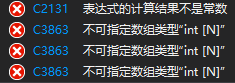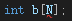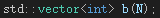## 2.归并排序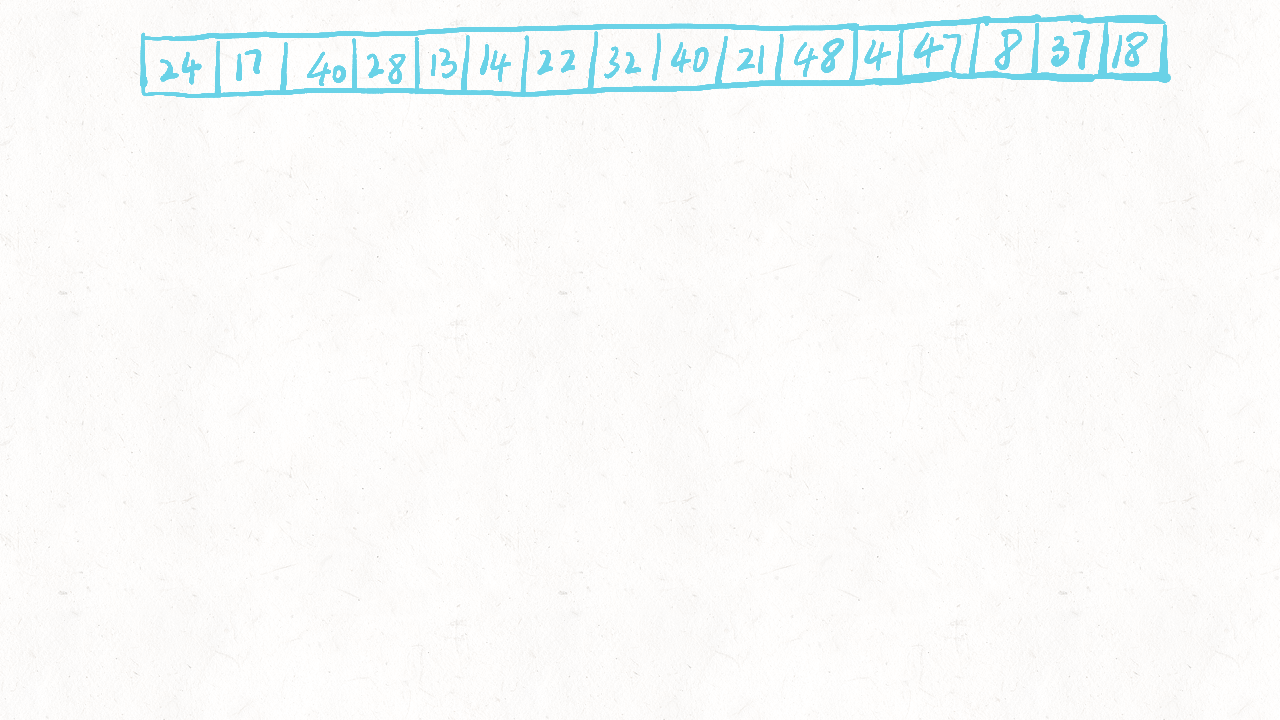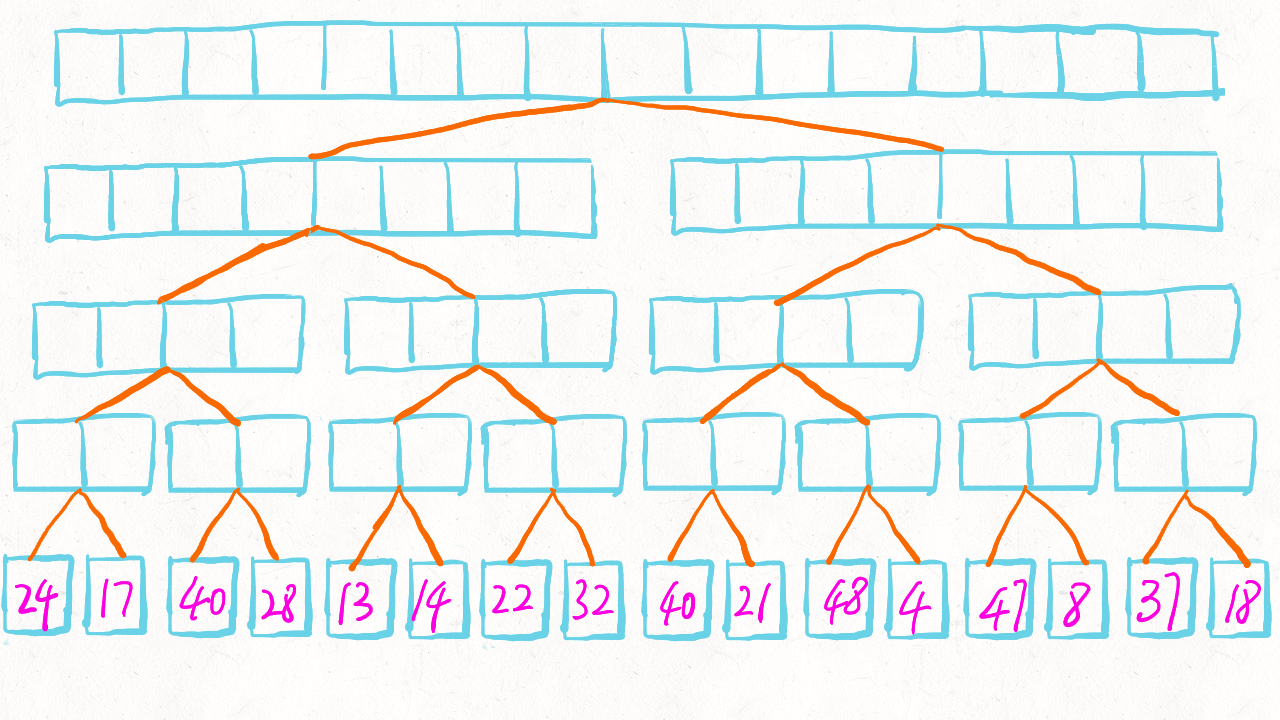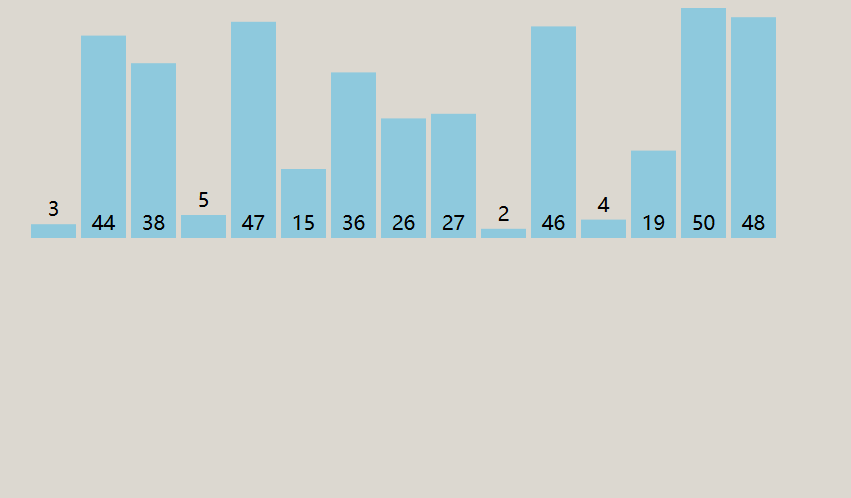### 2.1 归并排序的C++实现

``````void merge(int a[], int low, int mid, int high) {
int N = high - low + 1;
std::vector<int> b(N);
int left = low, right = mid + 1, bIdx = 0;
while (left <= mid && right <= high)
b[bIdx++] = (a[left] <= a[right]) ? a[left++] : a[right++];
while (left <= mid) b[bIdx++] = a[left++];
while (right <= high) b[bIdx++] = a[right++];
for (int k = 0; k < N; k++) a[low + k] = b[k];
}``````
``````void mergeSort(int a[], int low, int high) {
// 要排序的数组是 a[low..high]
if (low < high) {
int mid = (low+high) / 2;
mergeSort(a, low  , mid ); // 分成一半
mergeSort(a, mid+1, high); // 递归地将它们排序
merge(a, low, mid, high); // 解决: 归并子程序
}
}``````